# Riemann curvature bundle

This lives as a subbundle of: the symmetric square of the exterior square of the tangent bundle, viz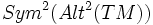$Sym^2(Alt^2(TM))$

## Definition

### Given data

A differential manifold$M$.

### Definition part

The Riemann curvature bundle on$M$ is the bundle whose fibre at every point in the Riemann curvature space at the point. It is a subbundle of the space of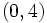$(0,4)$-tensor bundle comprising those tensors that satisfy the symmetry and antisymmetry conditions and the first Bianchi identity. Namely,$R$ is in the Riemann curvature space if at every point:

1.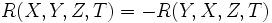$R(X,Y,Z,T) = -R(Y,X,Z,T)$ (viz$R$ is antisymmetric in the first two variables)
2.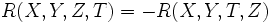$R(X,Y,Z,T) = -R(X,Y,T,Z)$ (viz$R$ is antisymmetric in the last two variables)
3.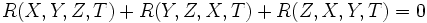$R(X,Y,Z,T) + R(Y,Z,X,T) + R(Z,X,Y,T) = 0$ (The first Bianchi identity)
4.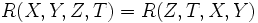$R(X,Y,Z,T) = R(Z,T,X,Y)$ (this follows from the above three)

## Facts

### Map from Riemannian metrics to sections of this bundle

Given any Riemannian metric$g$ on$M$, the Riemann curvature tensor corresponding to that lives as a section of the Riemann curvature bundle.

### Flows on the Riemann curvature bundle

Any flow of a metric gives a corresponding flow on the space of sections to the Riemann curvature bundle. Thus, to study the flow of the metric, we may be interested in also studying the corresponding flow on the Riemann curvature bundle.